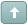Error

 Previous Topic Next Topic
MS Support
#1 Posted : Tuesday, November 5, 2019 5:55:19 PM(UTC)

Groups: Moderators, Registered, Registered Users, Subscribers
Joined: 10/8/2010(UTC)
Posts: 1,588

Thanks: 50 times
Was thanked: 125 time(s) in 121 post(s)

Markos Katsanos’ article, “The Stiffness Indicator,” introduces an indicator of the same name. He also included an exploration and an optimized system test using the indicator. The formulas to put these into MetaStock are shown below:

# Stiffness Indicator

Code:
``````tp:= Input("Stiffness Period", 2, 1000, 60);
MAB:= Input("Moving Average Period", 2, 1000, 100);
SM:= Input("Smooth Coeff.",1,20,3);
STIFFCRIT:= Input("STIFFCritical",70,100,90);
NSTD:= Input("Min SD",0,2,.2);
MA2:= Mov(C,MAB,S)-NSTD*Stdev(C,MAB);
PENS:= Sum(C>MA2, tp);
STIF:= PENS*100/ tp;
Mov(STIF,SM, E);
STIFFCRIT``````

# Stiffness Strategy

SYSTEM TEST NOTES

21 day inactivity stop suggested

4 optimization variables are used. Suggested values are:

Opt1 - Moving Average periods - suggested range: 50-120 with a step of 10

Opt2 - STIFF periods - suggested range of 40-80 with a step of 10

Opt3 - STIFFCRIT - suggested range of 90-95 with a step of 5

Opt4 - STIFFSELL - suggested range of 50-60 with a step of 10

Code:
``````MAB:= Opt1; {MA days: suggested range of 50-120 with a step of 10}
tp:= Opt2; {STIFF PERIOD: suggested range of 40-80 with a step of 10}
STIFFCRIT:= Opt3; {STIFFCRIT: suggested value of 90 or 95}
s1:= Security("ONLINE:SPY", C);
MA2:= Mov(C,MAB,S) - (0.2*StDev(C,MAB));
PENS:= Sum(C>MA2, tp);
STIF:= PENS*100/ tp;
STIFFNESS:= Mov(STIF,3, E);
Mov(s1,100,E)>=Ref(Mov(s1,100,E),-2) AND Cross(STIFFNESS,STIFFCRIT)``````

Sell Order

Code:
``````MAB:= Opt1; {MA days: suggested range of 50-120 with a step of 10}
tp:= Opt2; {STIFF PERIOD: suggested range of 40-80 with a step of 10}
STIFFSELL:= Opt4; {STIFFSELL: suggested value of 50 or 60}
MA2:= Mov(C,MAB,S) - (0.2*StDev(C,MAB));
PENS:= Sum(C>MA2, tp);
STIF:= PENS*100/ tp;
STIFFNESS:= Mov(STIF,3, E);
Cross(STIFFSELL,STIFFNESS)``````

STOPS

Inactivity Minimum Change:

Positions: Longs

Method: Percent

Minimum Change: 100

Periods: 21

OPTIMIZATIONS

OPT1

Description: MA Periods

Minimum: 50

Maximum: 120

Step: 10

OPT2

Description: STIFF Periods

Minimum: 40

Maximum: 80

Step: 10

OPT3

Description: STIFF crit

Minimum: 90

Maximum: 95

Step: 5

OPT4

Description: STIFF sell

Minimum: 50

Maximum: 60

Step: 10

# Stiffness Exploration

EXPLORATION NOTES

Columns reported are:

1- Current Price

2- Current Volume

3- Volume divided by Average Volume

4- RSI

5- Stiffness

6- Profit Target

COLUMN FORMULAS

Column A

Column Name: Price

Formula:

Code:
``C``

Column B

Column Name: Volume

Formula:

Code:
``VOLUME``

Column C

Column Name: V / AvgV

Formula:

Code:
``````ma1:= Mov(V,2,S);
ma2:= Mov(V,50,S);
denom:= If(ma2=0, -1, ma2);
If(denom=-1, 0, Mov(V,2,S)/denom)``````

Column D

Column Name: RSI

Formula:

Code:
``RSI(6)``

Column E

Column Name: Stiffnes

Formula:

Code:
``````MAB:= 100; {Moving Average periods}
tp:= 60; {STIFFNESS PERIOD}
STIFFCRIT:= 90; {STIFFNESS CRITICAL}
MA2:= Mov(C,MAB,S) - (0.2*Stdev(C,MAB));
PENS:= Sum(C>MA2, tp);
STIF:= PENS*100/ tp;
Mov(STIF,3, E)``````

Column F

Column Name: target

Formula:

Code:
``C + ( 8*ATR(50) )``

EXPLORATION FILTER

Formula:

Code:
``````MAB:= 100; {Moving Average periods}
tp:= 60; {STIFFNESS PERIOD}
STIFFCRIT:= 90; {STIFFNESS CRITICAL}
s1:= Security("ONLINE:SPY", C);
MA2:= Mov(C,MAB,S) - (0.2*Stdev(C,MAB));
PENS:= Sum(C>MA2, tp);
STIF:= PENS*100/ tp;
STIFFNESS:= Mov(STIF,3, E);
Mov(s1,100,E)>=Ref(Mov(s1,100,E),-2) AND Cross(STIFFNESS,STIFFCRIT)``````

 Wanna join the discussion?! Login to your Discussions forum accountor Register a new forum account.Users browsing this topic
Forum Jump
You cannot post new topics in this forum.
You cannot reply to topics in this forum.
You cannot delete your posts in this forum.
You cannot edit your posts in this forum.
You cannot create polls in this forum.
You cannot vote in polls in this forum.